# Essential Excel Skills For Researchers Part 2

Jeff Sauro, PhDExcel is an invaluable tool for analyzing and displaying data. In Part 1 I covered some essentials Excel skills, such as conditionals, absolute references and the fill handle.

In this second part I’ll cover a few more advanced functionalities that mimic database manipulations. You can also find these examples in the downloadable spreadsheet.

## 1. VLOOKUP

VLOOKUPs separate the novice from the intermediate Excel analyst. A VLOOKUP joins variables from two tables in a database without using SQL. For example, you’ll often have a list of variables that you need to connect with another list. In the figure below, the Class Mean on a 7-point scale is provided in column B. The value for each class needs to then be added to column G.  So for example, cell G3 needs to be a 5, G5 a 2.2 and so forth.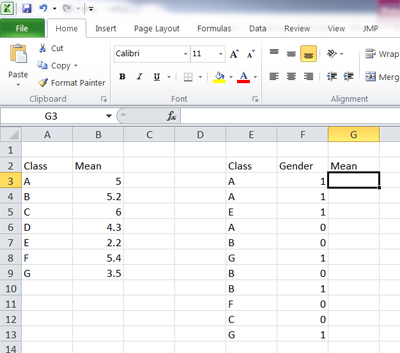To do this using a VLOOKUP you need to specify the value you want to match and where the source data is in the spreadsheet. Here is the final formula we will use = VLOOKUP(E3,\$A\$3:\$B\$9,2,FALSE).

Here’s how you build the formula for this example

• The value you want to “look up” is in column E and the first cell is E3. Start the formula in cell G3 and drag down.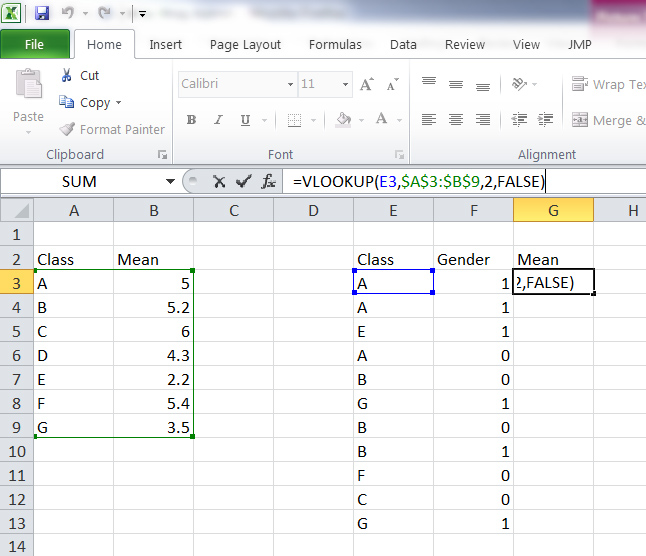• Specify the location of the values table. The lookup value needs to be in the left-most column. The lookup table is between cells \$A\$3:\$B\$9 (the green box in the figure below). The dollar signs are the absolute reference from part 1
• The next parameter in the formula is the column you want to return, which is the second column in the source table (green box).
• The FALSE in the formula means Excel won’t do a partial match (which sometimes you want to do, but usually not). The results are shown below.## 2. Sum/Count/Average in the corner

Because counting and averaging and adding are so common in Excel, Excel has a shortcut many people don’t know about. Just highlight the cells you have values in and look in the lower-right corner and Excel displays a count, total, and average!## 3. Error Bars

Error bars are what Excel calls the function to add confidence intervals to a graph. Here’s how to display the error bars:

1. With a bar graph created and the confidence interval values generated, select the Error Bars option, which is on the Ribbon (or some similar location depending on your version of Excel).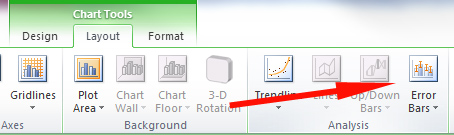2. From the Format Error Bars dialog, select the Custom radio button and then the Specify Value button.3. For confidence intervals with symmetrical margins of errors (like rating scales), select the cell that contains the margin of error (.76 in this example) for both the Positive Error Value and Negative Error Value as shown in the figure below.4. Click OK on both dialogues and you’ll then get your confidence intervals displayed.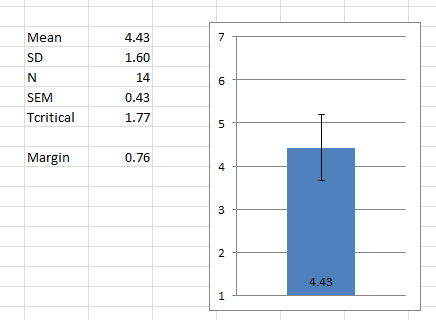For displaying asynchronous confidence intervals, such as for the Adjusted Wald Confidence Interval, you need to specify different Positive and Negative Error values.

1. Create a Graph Low and Graph High Value by subtracting your percentage from the lower end of the 90% confidence interval (Graph Low Cell) and subtract the percentage from the 90% high value (Graph High Cell) as shown in the figure below.2. Similar to the steps above, point the Positive Error Value to the new Graph High cell value (10% in this case) and point the Negative Error Value to the Graph Low cell value (13% in this case).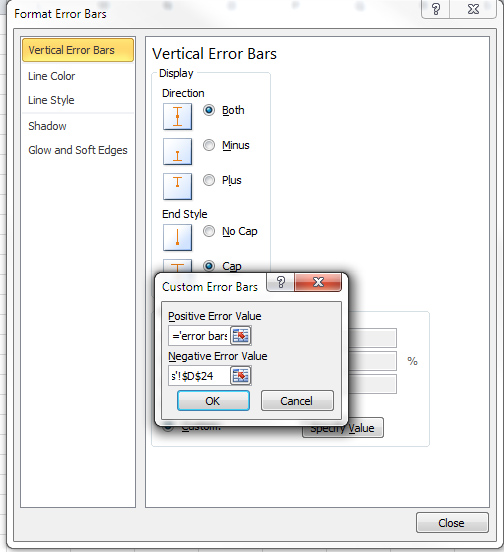3. You’ll now get the confidence intervals on your bar graph as shown in the figure below.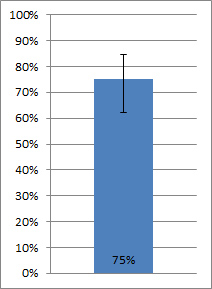## 4. Pivot table

You could write a book on Pivot tables. Pivot tables are basically cross-tabs for Excel. For example, you might want to know what the breakdown of gender by education is from an output of data. Instead of setting up a bunch of counts and IF statements, use a pivot table. I have a dataset with categories (0 = men and women = 1) and a set of categories for education as shown in the figure and example spreadsheet.

1.    Click the Pivot Table button on the Ribbon.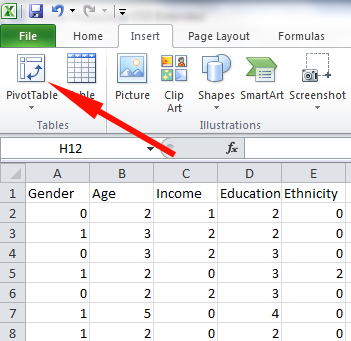2.    Select the columns that contain your data. You must have labels at the top and no spaces.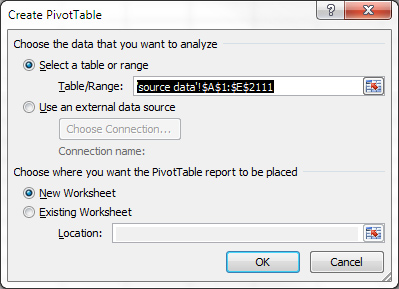In this example, my data source is from columns A to E on Sheet 2. It places the table in a new sheet by default.
You’ll get a screen that looks something like the following: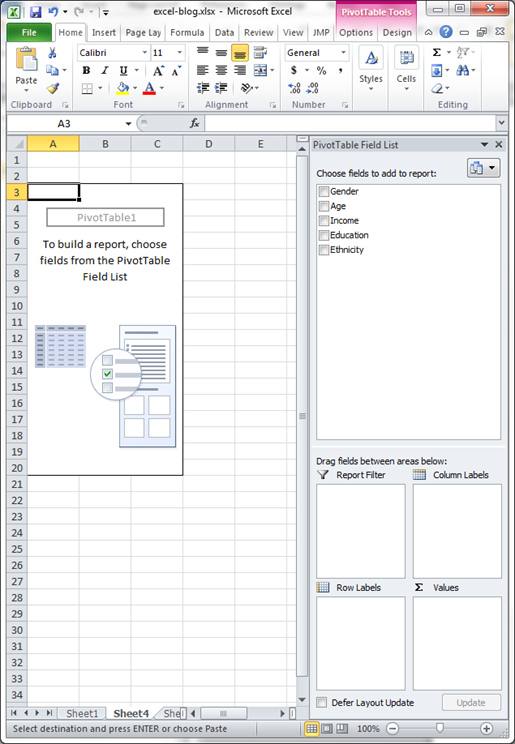3.    Drag and drop Gender into the Values field and Education in the Row Labels field. Then drop Gender again in the Column Labels field. This shows you the count of gender for each level of education.  The drop-down in the Values field can be changed to a number of operations including Average and Sum.## 5. Autofilter

You can do another database action with one click of the button. Highlight your data with column headings and select the Filter icon on the Ribbon. This turns your static column into a dynamic database.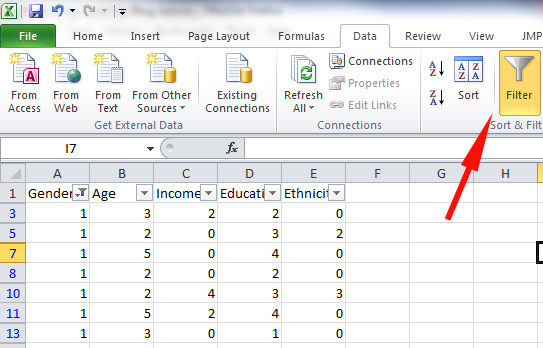You can then see only the data from women by selecting “1” from the column’s drop-down list.0
0